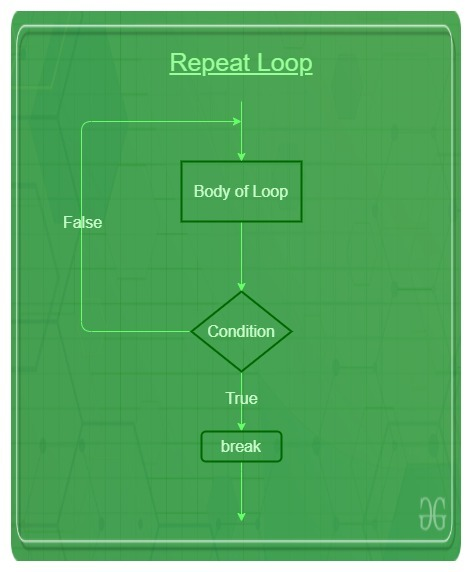# R – Repeat loop

• Last Updated : 21 Apr, 2020

Repeat loop in R is used to iterate over a block of code multiple number of times. And also it executes the same code again and again until a break statement is found.

Repeat loop, unlike other loops, doesn’t use a condition to exit the loop instead it looks for a break statement that executes if a condition within the loop body results to be true. An infinite loop in R can be created very easily with the help of the Repeat loop. The keyword used for the repeat loop is `'repeat'`.

Syntax:

```repeat {
commands
if(condition) {
break
}
}```

Flowchart:Example 1:

 `# R program to illustrate repeat loop`` ` `result <``-` `c(``"Hello World"``)``i <``-` `1`` ` `# test expression ``repeat {`` ` `   ``print``(result)``    ` `   ``# update expression ``   ``i <``-` `i ``+` `1``    ` `   ``# Breaking condition``   ``if``(i >``5``) {``      ``break``   ``}``}`

Output:

``` "Hello World"
 "Hello World"
 "Hello World"
 "Hello World"
 "Hello World"```

Example 2:

 `# R program to illustrate repeat loop`` ` `result <``-` `1``i <``-` `1`` ` `# test expression ``repeat {`` ` `   ``print``(result)``    ` `   ``# update expression ``   ``i <``-` `i ``+` `1``   ``result ``=` `result ``+` `1`` ` `   ``# Breaking condition``   ``if``(i > ``5``) {``      ``break``   ``}``}`

Output:

``` 1
 2
 3
 4
 5```

My Personal Notes arrow_drop_up